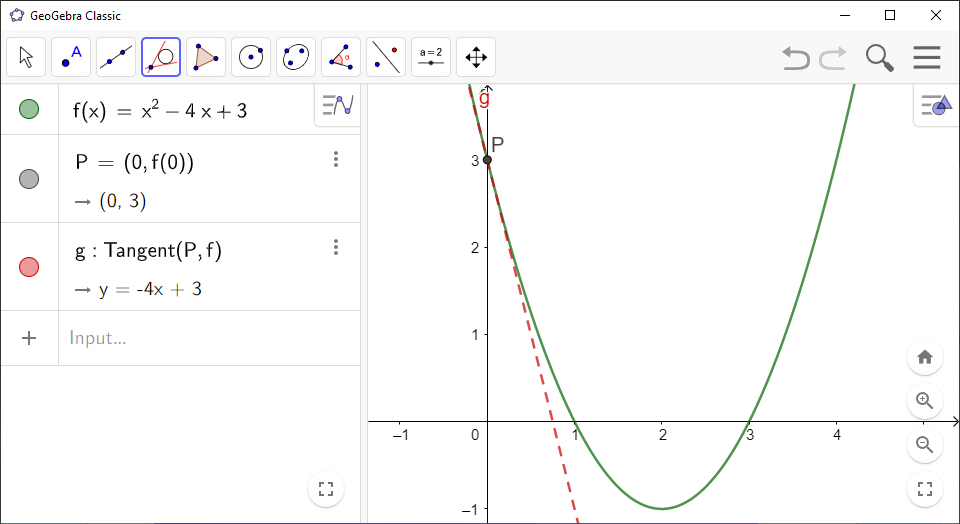# How to Draw a Tangent to a Curve in GeoGebra

You can use `GeoGebra` to find the tangent line to the graph of a function at a given point:

`GeoGebra` Instruction 1

### TheTangentataPoint

1.
Open `Algebra View` and `Graphics View` under`View` in`Menu`.
2.
Type the function in `Algebra View` and press `Enter`.
3.
Enter the point $\left({x}_{1},{y}_{1}\right)$ in `Algebra View` and press `Enter`. Her ${y}_{1}=f\left({x}_{1}\right)$.
4.
Select the tool `Tangents`in the `Toolbar`, click the point followed by the graph you have made. The equation for the tangent will appear in `Algebra View`.